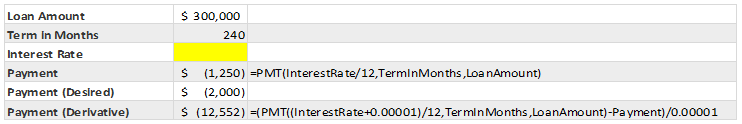## What is Goal Seek?

In numerical analysis, goal seek constitutes a question: under a given set of criteria, what degree of change is required to achieve a convergence between the actual and a theoretical result? It can be understood as a tool for determining the cause and effect of manipulating certain pieces of input data until the desired output is achieved.

## The Math behind Goal Seek

The means by which we can achieve this type of analysis is a method of recursion, known as the Newton-Raphson method. This iterative technique follows a set guideline to approximate one root (considering the function), its derivative, and an initial starting value.In the context of a spreadsheet-based application, the function above (f(x)) is representative of the entire series of calculations performed in the Excel document. The initial x-value is chosen as the starting point for all input to this function – and thus, to the Excel-based calculations. The iterations over the function (or Excel-based calculations) will begin recursive execution starting with this set of input criteria (x).

The following function is representative of determining the derivative of the Excel document’s cumulative calculations:Effectively, this formula of differentiation states that the function must be run two times and the difference between the y values (or output) should be divided by the difference between the x values (or input), resulting in our derivative – which the sensitivity to change of one value (y) with respect to another value (x).

Note: For an accurate measurement, the difference between the x values, or independent variable, should be small – in our subsequent examples, we will be using 0.00001.

Once your spreadsheet has been uploaded to the SpreadsheetWEB server, either as a web service or web page, we can achieve the Newton-Raphson approach in one of two ways:

1. We can call the SpreadsheetWEB API for both x values, performing the differential calculation on the web; however, this approach will unnecessarily double the load on the engine for each individual step of iteration.
2. We can find the derivative value in separate cell in Excel and request, both, the actual results and the derivative with a single API call.

We will be demonstrating the second approach since it is more efficient programmatically.

## Implementing Goal Seek Programmatically

The iterative process of the Newton-Raphson method is replicated by recursive calls to a function that will process the spreadsheet calculations on the server and return the expected results with each iteration.The iterative structure is set up as follows:

1. We set up all starting criteria and target values.
2. We define the inputs and outputs (I/O) that the API will use when calling the server to perform the calculations (e.g. in this spreadsheet, there would be an input named “x” and two outputs named “Fx” and “F_x”).
3. We define the GetResultRecur function, which will be used to recursively call the SpreadsheetWEB API. The callback function will handle the results, either concluding the recursion or continuing it based on the maximum iterations and a comparison between the tolerance and target value.

This specific workflow goes as follows:

1. The GetResult function of the API is executed with the initial x value, requesting Fx and F_x as output from the API.
2. Once the API returns the output, the callback function will map the results of Fx and F_x to the Fx and F_x variables, respectively.
3. Xn1 is calculated as the differential (Newton-Raphson method).
4. If the differential (Xn1) meets the tolerance, then the solution has been found and the function is concluded.
5. If the tolerance has not been met, then the new starting value (x) is set to the result of the prior iteration (Xn1), the inputs are reset accordingly, and the API is called again for the next iteration.

## Target Interest Rate with User Defined Payments

Calculating the target interest rate on a loan with a user defined payment structure exemplifies a practical use case for the goal seek calculation approach. Creating a web application that performs these types of calculations is relatively simple using the SpreadsheetWEB API.

Let’s take a particular example of a user wanting to borrow \$300,000, aiming to pay off the loan over the course of 240 months, capable of paying \$2000 per month. A simple PMT function (calculating the monthly payment amount) can also be used to calculate the target interest rate via recursion. In this scenario, the goal you are seeking is the monthly payment amount.

The Excel spreadsheet may look something like this:Our goal is find the interest rate which constitutes a monthly payment of \$2000. While the input cell is empty (named InterestRate), both the original function and its derivative are calculated in Excel – these inputs are named Payment and PaymentDer, respectively.

In the web application, we will simply be finding the interest rate, sending it to the Excel file, retrieving the payment values, and determining whether or not the goal has been achieved. This is identical to the form and structure of the prior code.

Note: the PMT function requires negative values for payments.A sample SpreadsheetWEB application implementing this code is below: